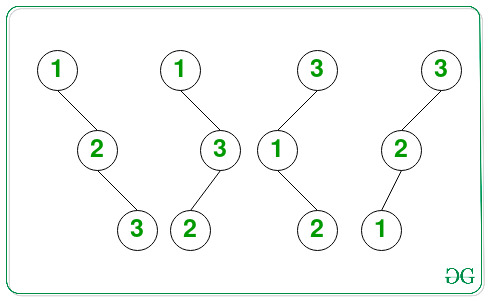Open In App

# Number of Binary Search Trees of height H consisting of H+1 nodes

Given a positive integer H, the task is to find the number of possible Binary Search Trees of height H consisting of the first (H + 1) natural numbers as the node values. Since the count can be very large, print it to modulo 109 + 7.

Examples:

Input: H = 2
Output: 4
Explanation: All possible BSTs of height 2 consisting of 3 nodes are as follows:Therefore, the total number of BSTs possible is 4.

Input: H = 6
Output: 64

Approach: The given problem can be solved based on the following observations:

• Only (H + 1) nodes are can be used to form a Binary Tree of height H.
• Except for the root node, every node has two possibilities, i.e. either to be the left child or to be the right child.
• Considering T(H) to be the number of BST of height H, where T(0) = 1 and T(H) = 2 * T(H – 1).
• Solving the above recurrence relation, the value of T(H) is 2H.

Therefore, from the above observations, print the value of 2H as the total number of BSTs of height H consisting of the first (H + 1) natural numbers.

Below is the implementation of the above approach:

## C++

 `// C++ program for the above approach` `#include ``using` `namespace` `std;` `const` `int` `mod = 1000000007;` `// Function to calculate x^y``// modulo 1000000007 in O(log y)``int` `power(``long` `long` `x, unsigned ``int` `y)``{``    ``// Stores the value of x^y``    ``int` `res = 1;` `    ``// Update x if it exceeds mod``    ``x = x % mod;` `    ``// If x is divisible by mod``    ``if` `(x == 0)``        ``return` `0;` `    ``while` `(y > 0) {` `        ``// If y is odd, then``        ``// multiply x with result``        ``if` `(y & 1)``            ``res = (res * x) % mod;` `        ``// Divide y by 2``        ``y = y >> 1;` `        ``// Update the value of x``        ``x = (x * x) % mod;``    ``}` `    ``// Return the value of x^y``    ``return` `res;``}` `// Function to count the number of``// of BSTs of height H consisting``// of (H + 1) nodes``int` `CountBST(``int` `H)``{` `    ``return` `power(2, H);``}` `// Driver Code``int` `main()``{``    ``int` `H = 2;``    ``cout << CountBST(H);` `    ``return` `0;``}`

## Java

 `// Java program for the above approach``class` `GFG{``    ` `static` `int` `mod = ``1000000007``;` `// Function to calculate x^y``// modulo 1000000007 in O(log y)``static` `long` `power(``long` `x, ``int` `y)``{``    ` `    ``// Stores the value of x^y``    ``long` `res = ``1``;` `    ``// Update x if it exceeds mod``    ``x = x % mod;` `    ``// If x is divisible by mod``    ``if` `(x == ``0``)``        ``return` `0``;` `    ``while` `(y > ``0``)``    ``{``        ` `        ``// If y is odd, then``        ``// multiply x with result``        ``if` `((y & ``1``) == ``1``)``            ``res = (res * x) % mod;` `        ``// Divide y by 2``        ``y = y >> ``1``;` `        ``// Update the value of x``        ``x = (x * x) % mod;``    ``}` `    ``// Return the value of x^y``    ``return` `res;``}` `// Function to count the number of``// of BSTs of height H consisting``// of (H + 1) nodes``static` `long` `CountBST(``int` `H)``{``    ``return` `power(``2``, H);``}` `// Driver code``public` `static` `void` `main(String[] args)``{``    ``int` `H = ``2``;``    ` `    ``System.out.print(CountBST(H));``}``}` `// This code is contributed by abhinavjain194`

## Python3

 `# Python3 program for the above approach` `# Function to calculate x^y``# modulo 1000000007 in O(log y)``def` `power(x, y):``    ` `    ``mod ``=` `1000000007``    ` `    ``# Stores the value of x^y``    ``res ``=` `1` `    ``# Update x if it exceeds mod``    ``x ``=` `x ``%` `mod` `    ``# If x is divisible by mod``    ``if` `(x ``=``=` `0``):``        ``return` `0``        ` `    ``while` `(y > ``0``):``        ` `        ``# If y is odd, then``        ``# multiply x with result``        ``if` `(y & ``1``):``            ``res ``=` `(res ``*` `x) ``%` `mod` `        ``# Divide y by 2``        ``y ``=` `y >> ``1` `        ``# Update the value of x``        ``x ``=` `(x ``*` `x) ``%` `mod``    ` `    ``# Return the value of x^y``    ``return` `res` `# Function to count the number of``# of BSTs of height H consisting``# of (H + 1) nodes``def` `CountBST(H):``    ` `    ``return` `power(``2``, H)` `# Driver Code``H ``=` `2` `print``(CountBST(H))` `# This code is contributed by rohitsingh07052`

## C#

 `// C# program for the above approach``using` `System;` `class` `GFG{``    ` `static` `int` `mod = 1000000007;` `// Function to calculate x^y``// modulo 1000000007 in O(log y)``static` `long` `power(``long` `x, ``int` `y)``{``    ` `    ``// Stores the value of x^y``    ``long` `res = 1;` `    ``// Update x if it exceeds mod``    ``x = x % mod;` `    ``// If x is divisible by mod``    ``if` `(x == 0)``        ``return` `0;` `    ``while` `(y > 0)``    ``{``        ` `        ``// If y is odd, then``        ``// multiply x with result``        ``if` `((y & 1) == 1)``            ``res = (res * x) % mod;` `        ``// Divide y by 2``        ``y = y >> 1;` `        ``// Update the value of x``        ``x = (x * x) % mod;``    ``}` `    ``// Return the value of x^y``    ``return` `res;``}` `// Function to count the number of``// of BSTs of height H consisting``// of (H + 1) nodes``static` `long` `CountBST(``int` `H)``{``    ` `    ``return` `power(2, H);``}` `// Driver code``static` `void` `Main()``{``    ``int` `H = 2;``    ` `    ``Console.Write(CountBST(H));``}``}` `// This code is contributed by abhinavjain194`

## Javascript

 ``

Output:

`4`

Time Complexity: O(log2H)
Auxiliary Space: O(1)